# Father and sons

Father is 27 and his sons 2 and one year. In how many years will his sons sum up to half his age?

Result

x =  7

#### Solution:

(2+x)+(1+x) = (27+x)/2

3x = 21

x = 7

Calculated by our simple equation calculator.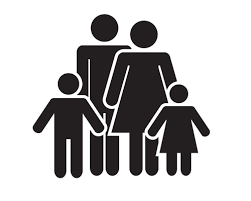Our examples were largely sent or created by pupils and students themselves. Therefore, we would be pleased if you could send us any errors you found, spelling mistakes, or rephasing the example. Thank you!

Leave us a comment of this math problem and its solution (i.e. if it is still somewhat unclear...):Be the first to comment!#### Following knowledge from mathematics are needed to solve this word math problem:

Looking for help with calculating arithmetic mean? Looking for a statistical calculator? Do you have a linear equation or system of equations and looking for its solution? Or do you have quadratic equation?

## Next similar math problems:

1. Father and son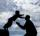Father is 44 years old, his son 16 years. Determine how many years ago was the father five times older than the son.
2. Holidaymakers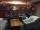A total of 94270 holidaymakers spent part of their holiday in 50 hotels a year. On average, how many vacationers are there for one hotel?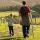Daddy is a 46 year old and son is at age of 16. When (which year) was/will daddy 5 times more years than his son?
4. Forest nurseryIn the forest nursery after winter, they found that 1/10 stems died out of them. For them, they land 193 new spruces. How many spruces are in the forest nursery?
5. The average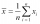The average of one set of 4 numbers is 35. The average of another set of number is 20. The average of the numbers in the two sets is 30. How many numbers are there in the other set?
6. Cakes1/3 poppy cake, 1/3 apple, 15 pieces of cheese. How many are totally cakes?
7. Apples 3Julka has 5 apples more than Hugo and four apples less than Annie. Hugo has 17 apples. How many apples has Julka and how Annie?
8. BenchesThe park has 64 benches. Occupied are by 18 more than empty. How many benches are occupied and empty ?
9. PopsiclesFrancis went to buy ice lollies. If he buy 8 popsicles he missed 4 USD. When he buy 7 popsicles, got back 1 USD. How many USD was a popsicle?
10. Hr to minSue biked to school in 5/12 of an hour. How many minutes did it take her to ride to school?
11. Eq1Solve equation: 4(a-3)=3(2a-5)
12. Simple equation 8Solve the following equation: 36=-(1+7x)-6(-7-x)
13. Simple equation 1035= 7*3*x what is x?
14. If-then equationIf 5x - 17 = -x + 7, then x =
15. Six te 2If 3t-7=5t, then 6t=
16. Temperature 2Sunday's high temperature was 3 degrees higher than Saturday's. On Monday, the temperature fell 5 degrees, then rose 7 degrees on Tuesday and 4 more on Wednesday. Then it fell 17 degrees to a record low of 31 on Thursday. What was the temperature on SaturdAdded together and write as decimal number: LXVII + MLXIV